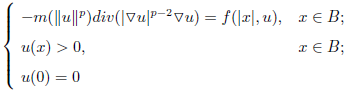# 带有非负局部项的p-Laplacian问题的径向正解Radial Positive Solutions of p-Laplacian Problem with Nonnegative Local Terms

DOI: 10.12677/AAM.2019.88166, PDF, HTML, 下载: 303  浏览: 388  科研立项经费支持Abstract:

In this paper, we mainly study the existence of radial solutions for the following p- Laplacian problem:where f and m satisfy certain conditions. We prove that the above p-Laplacian problem has a radial solution through the origin mainly by means of upper and lower solutions. Firstly, we make the  auxiliary  problem  sequence  of  the  original  problem.  Then  we get a monotone bounded solution sequence by solving the problem sequence. Then we can get that when n tends to infinity, there exists a u, which makes this solution sequencetend to u. Finally, we prove that u is the radial solution of  the  original problem.  The concrete proof is given in the third part.

  Santos Jr., J.R. and Siciliano, G. (2018) Positive Solutions for a Kirchhoff Problem with Vanishing Nonlocal Term. Journal of Differential Equations, 265, 2034-2043. https://doi.org/10.1016/j.jde.2018.04.027  Le, P., Huynh, N. and Ho, V. (2019) Positive Solutions of the p-Kirchhoff Problem with Degenerate and Sign-Changing Nonlocal Term. Zeitschrift fu¨r Angewandte Mathematik und Physik, 70, 68. https://doi.org/10.1007/s00033-019-1114-2  Chu, K., Hai, D. and Shivaji, R. (2019) Uniqueness of Positive Radial Solutions for Infinite Semipositone p-Laplacian Problems in Exterior Domains. Journal of Mathematical Analysis and Applications, 472, 510-525. https://doi.org/10.1016/j.jmaa.2018.11.037  Lu¨, H. and Bai, Z. (2006) Positive Radial Solutions of a Singular Elliptic Equation with Sign Changing Nonlinearities. Applied Mathematics Letters, 19, 555-567. https://doi.org/10.1016/j.aml.2005.08.002  Jin, C.H., Yin, J. and Wang, Z. (2007) Positive Radial Solutions of p-Laplacian Equation with Sign Changing Nonlinear Sources. Mathematical Methods in the Applied Sciences, 30, 1-14. https://doi.org/10.1002/mma.771  夏道行, 严绍宗, 舒五吕, 等. 泛函分析第二教程[M]. 北京: 高等教育出版社, 2008.  Habets, P. and Zanolin, F. (1994) Upper and Lower Solutions for a Generalized Emden-Fowler Equation. Journal of Mathematical Analysis and Applications, 181, 684-700. https://doi.org/10.1006/jmaa.1994.1052